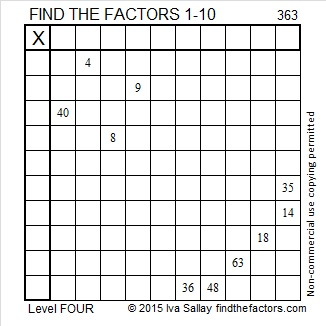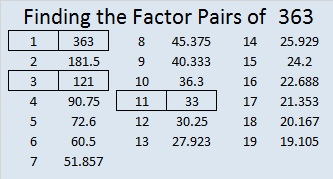# 363 and Level 4

Every digit of 363 is divisible by 3, so 363 is divisible by 3 and is a composite number. Its factor information is given below the puzzle.Print the puzzles or type the factors on this excel file: 10 Factors 2015-01-19

• 363 is a composite number.
• Prime factorization: 363 = 3 x 11 x 11, which can be written 363 = 3 x (11^2)
• The exponents in the prime factorization are 1 and 2. Adding one to each and multiplying we get (1 + 1)(2 + 1) = 2 x 3  = 6. Therefore 363 has exactly 6 factors.
• Factors of 363: 1, 3, 11, 33, 121, 363
• Factor pairs: 363 = 1 x 363, 3 x 121, or 11 x 33
• Taking the factor pair with the largest square number factor, we get √363 = (√3)(√121) = 11√3 ≈ 19.053